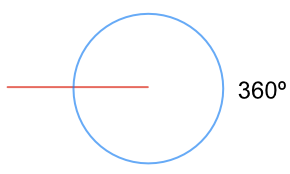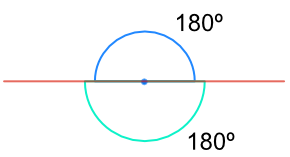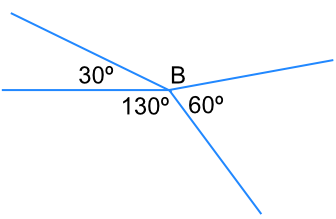Angles at a Point

## Angles at a Point

Angles about a point add up to 360º (which is a complete circle).If a point is on a straight line, then the angles on each side of the line add up to 180º.## Example 1

What is the value of the angle A in this diagram?There are 360º in a circle. 140º has been allocated to one angle.

There are 360-140 = 220º left in the circle.

## Example 2

What is the value of the angle, B, in the diagram below?Angles at a point add up to 360º.

Adding up the known angles: 30 + 130 + 60 = 220º.

Subtract from 360º: 360 - 220 = 140º.